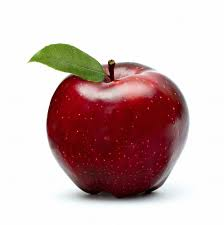# I am apple @_@

Probability Level 3There are $4$ types of boxes of $\color{#D61F06}{\mathrm{apples}}$,

$\bullet \quad$ boxes containing $40$ $\color{#D61F06}{\mathrm{apples}}$,

$\bullet \quad$ boxes containing $16$ $\color{#D61F06}{\mathrm{apples}}$,

$\bullet \quad$ boxes containing $56$ $\color{#D61F06}{\mathrm{apples}}$,

$\bullet \quad$ boxes containing $32$ $\color{#D61F06}{\mathrm{apples}}$,

Then find the maximum number of $\color{#D61F06}{\mathrm{apples}}$ less than $2014$ which can be given by giving some boxes of some of the types stated above.

Details and assumptions:-

You are allowed to give 0 boxes of a certain type(s), and thus, find the maximum number.

This problem is a part of the set Vegetable Combinatorics , try other problems too !

×